Cart
× This is a Sterling guide price. You will be invoiced in the appropriate currency for your country.
• ISBN:9781316600238
• Format:Cambridge Elevate
• Subject(s):Mathematics
• Qualification:Cambridge AS and A Level
• Author(s):Hugh Neill, Douglas Quadling, Julian Gilbey
• Available from: March 2017

Cambridge AS and A Level Mathematics is a revised series to ensure full syllabus coverage.

× This is a Sterling guide price. You will be invoiced in the appropriate currency for your country.

Send a Query

formRow
×
• DESCRIPTION

This Cambridge Elevate edition coursebook meets the requirements for the Pure Mathematics 2 and 3 (P2 and P3) units of Cambridge AS and A Level Mathematics (9709). Each chapter provides students with clear learning objectives, and mathematical concepts are clearly and carefully explained throughout. Worked examples take a step-by-step approach to problem solving and key results are highlighted in boxes for easy reference. Pure Mathematics 2 & 3 corresponds to units P2 and P3. It covers algebra, logarithmic and exponential functions, trigonometry, differentiation, integration, numerical solution of equations, vectors, differential equations and complex numbers.

• Contents
• FEATURES
• Reviewed and revised content to ensure most up-to-date syllabus coverage.

• Full endorsement from Cambridge International Examinations.

• Fully updated review questions to better reflect the type of questioning in the AS and A Level Mathematics qualification.

• Redesigned materials to keep content engaging.

• Learning objectives at the start of each chapter.

• Clear, narrative explanation of mathematical concepts, terminology and notation, with results and procedures appearing in boxes for easy reference.

• Stimulating worked examples that take a step-by-step approach to problem solving.

• CONTENTS
• Introduction
• 1. Polynomials
• 2. The modulus function
• 3. Exponential and logarithmic functions
• 4. Differentiating exponentials and logarithms
• 5. Trigonometry
• 6. Differentiating trigonometric functions
• Revision exercise 1
• 7. Differentiating products
• 8. Solving equations numerically
• 9. The trapezium rule
• 10. Parametric equations
• 11. Curves defined implicitly
• Revision exercise 2
• Practice exam-style papers for P2
• 12. Vectors: lines in two and three dimensions
• 13. Vectors: planes in three dimensions
• 14. The binomial expansion
• 15. Rational functions
• 16. Complex numbers
• 17. Complex numbers in polar form
• 18. Integration
• 19. Differential equations
• Revision exercise 3
• Practice exam-style papers for P3
• Index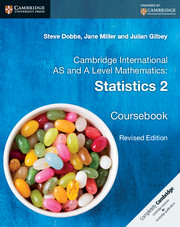£17.50 (+VAT) Cambridge International AS and A Level Mathematics: View product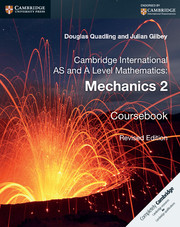£18.75 (+VAT) Cambridge International AS and A Level Mathematics: View product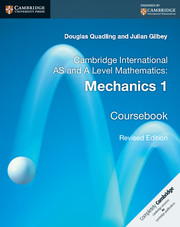£18.75 (+VAT) Cambridge International AS and A Level Mathematics: View product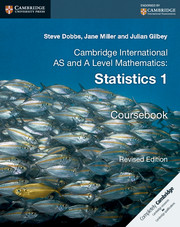£15.25 (+VAT) Cambridge International AS and A Level Mathematics: View product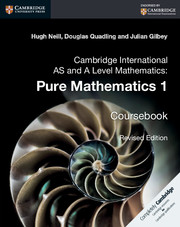£18.75 (+VAT) Cambridge International AS and A Level Mathematics: View product

Not already registered? Create an account now. ×

Other Cambridge websitesNeed a local sales consultant?